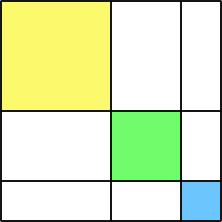#### You may also like### Areas and Ratios

Do you have enough information to work out the area of the shaded quadrilateral?### Shape and Territory

If for any triangle ABC tan(A - B) + tan(B - C) + tan(C - A) = 0 what can you say about the triangle?### Napoleon's Hat

Three equilateral triangles ABC, AYX and XZB are drawn with the point X a moveable point on AB. The points P, Q and R are the centres of the three triangles. What can you say about triangle PQR?

# Look Before You Leap

##### Age 16 to 18Challenge Level

If $a+b+c=4$,
$ab+bc+ca=6$
and $abc=3$,

what are the values of:
${1\over a}+ {1\over b }+ {1\over c}$ (think of fractions),

${1\over ab}+ {1\over bc }+ {1\over ca}$

and $a^2 +b^2 + c^2$?In the diagram the coloured squares have sides of length $a$, $b$ and $c$. Use the areas in the diagram to write down a formula for the expansion of $(a + b + c)^2$ and explain your method.

Using your expansion of $(a + b + c)^2$ to help you, expand $(a + b + c)^3$. Can you explain each term of the expansion using a diagram of a cube where each face has been cut up in a similar way to the square above?